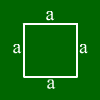# Square calculationCalculate a square Enter one value. Other values will be calculated. Side Area: Perimeter: diagonal
Enter the coordinates of for points. Mathepower calculates what (if any) quadrangle they form
A(||)

B(||)

C(||)

D(||)

The following formulas hold for squares:
Area = side * side
perimeter = side * 4
diagonal = square root of (side^2*side^2) (due to Pythagoras)

## What is a square?This is a square. A square is a quadrangle having four sides of the same length and perpendicular to each other. This means, a square is a rectangle and a rhomb at the same time.
In a square, these formulas hold: The area equals a*a.
The diagonal has the length a*(square root of 2).

For further information move to mouse over one of the words below and the corresponding part of the square will be marked.side a
perimeter
diagonal
area